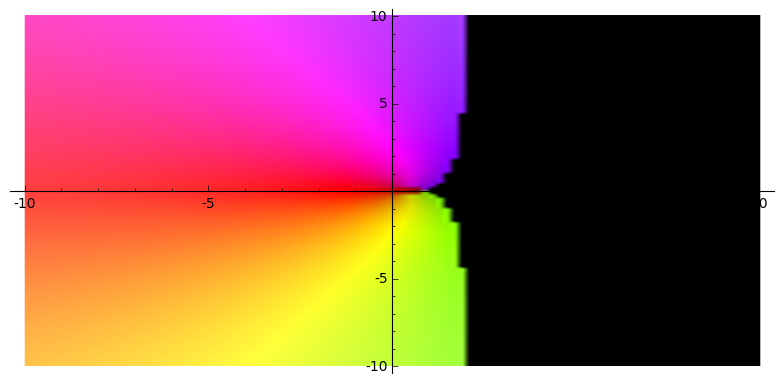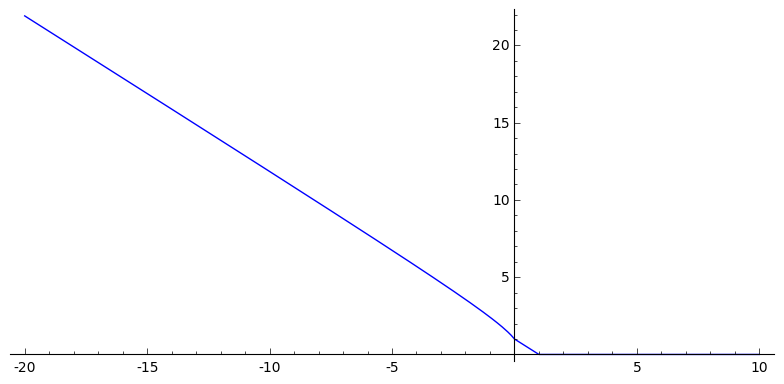# How do I plot the magnitude of a complex-valued function?

My script is like this

 v = var('v')

 assume(v,'complex') G = matrix([[0,1,0,0],[-v,1-v,0,0],[0,-v,1-v,0],[0,0,1,0]]) eigs = G.eigenvalues() f1(v) = abs(eigs) plot(f1,(-1,7))

The first eigenvalue is complex in a certain region and real elsewhere. But it does not plot those portions of the plot where the eigenvalue becomes complex. What gives?

My plot is broken where the eigenvalue is complex, with the error:

verbose 0 (2717: plot.py, generate_plot_points) WARNING: When plotting, failed to evaluate function at 140 points. verbose 0 (2717: plot.py, generate_plot_points) Last error message: 'math domain error'

What I want to do is observe the behavior of the magnitude of the eigenvalue with respect to v, irrespective of whether the eigenvalue is real or complex.

If I try  G = matrix(CC, [[0,1,0,0],[-v,1-v,0,0],[0,-v,1-v,0],[0,0,1,0]]) , I get an error.

edit retag close merge delete

Sort by » oldest newest most votedPerhaps this is what you want to use.

complex_plot(lambda v: abs(matrix(CC, [[0,1,0,0],[-v,1-v,0,0],[0,-v,1-v,0],[0,0,1,0]]).eigenvalues()),(-10,10),(-10,10))


Sample output:Although, then you may want to drop the abs to get:If you are just interested in the case where $v\in \mathbb{R}$ and want to plot the module of the eigenvalue then

plot(lambda v: abs(matrix(CC, [[0,1,0,0],[-v,1-v,0,0],[0,-v,1-v,0],[0,0,1,0]]).eigenvalues()),(x,-20,10))


will do the job.Update: Added case where $v\in\mathbb{R}$.

more

For further details about complex_plot you can consult the manual.

Thanks for the reply! You're right, I don't want v to be complex, but I thought that might force the eigenvalues to be interpreted as complex, or something. What I need is - how the magnitude of the eigenvalue behaves w.r.t. v, irrespective of whether the eigenvalue is real or complex.

You may need to use a lambda function.

lambda eg: abs(eg)


Haven't tested but some variant on this should work.

more